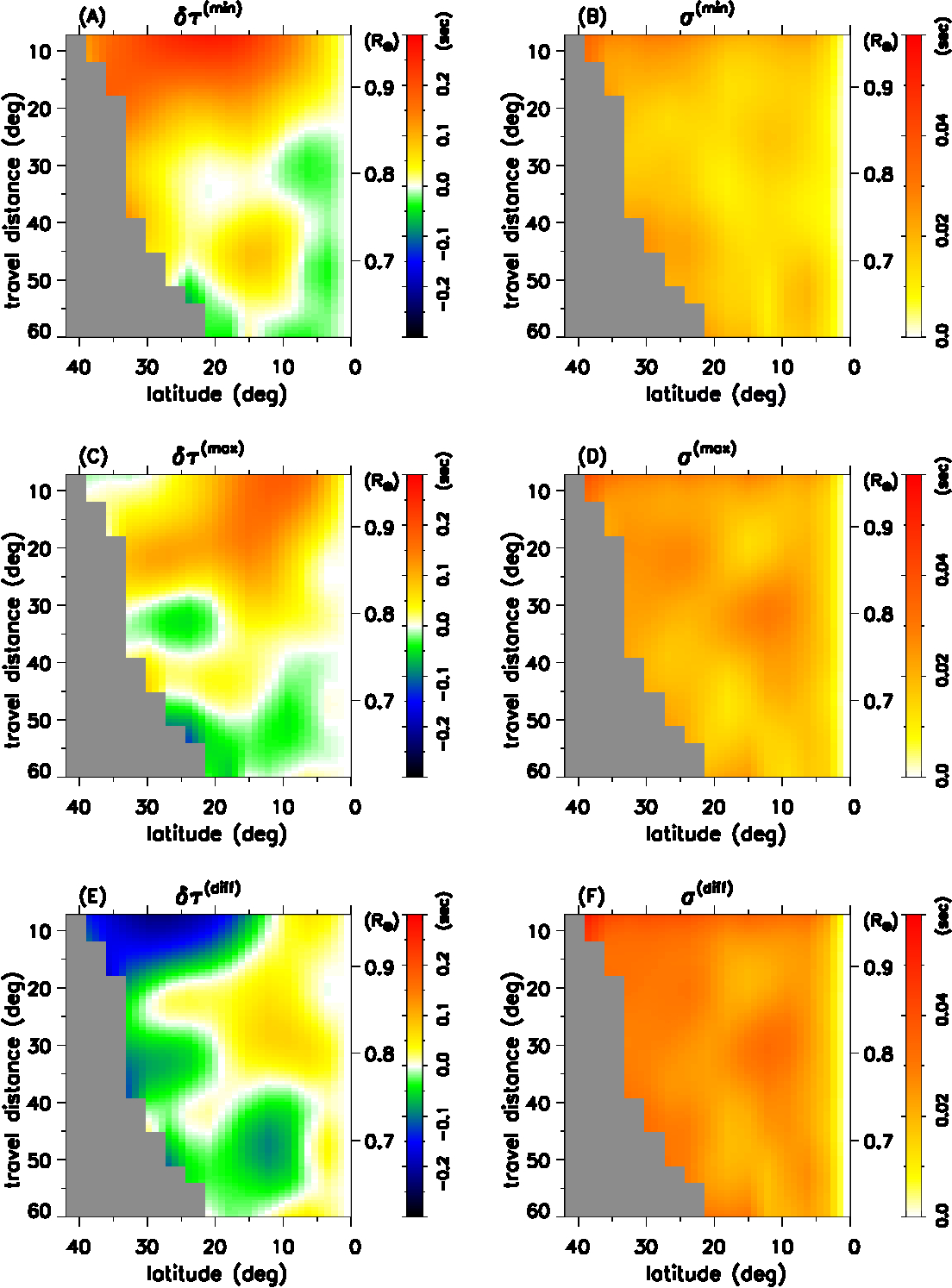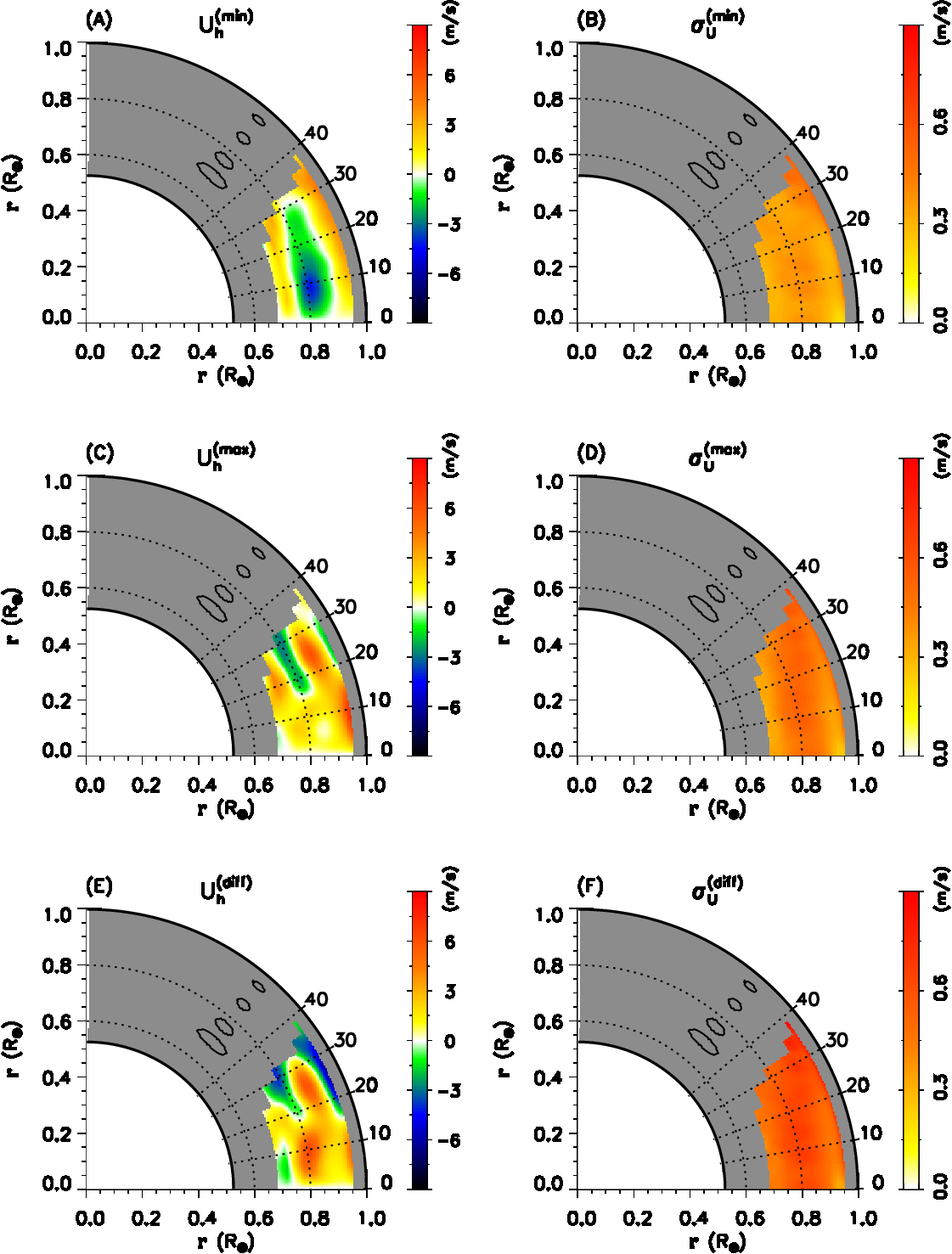# 98. Solar-Cycle Variations of Meridional Flows in the Solar Convection Zone with Helioseismic Methods

Contributed by Chia-Hsien Lin. Posted on May 18, 2018

Chia-Hsien Lin1 & Dean-Yi Chou2
1 Graduate Institute of Space Science,National Central University, Taiwan
2 Department of Physics, National Tsing-Hua University, Taiwan

Solar magnetic fields play a dominant role in most of observed solar activity. How and where the magnetic fields are generated has been one of the most important issues in the solar physics. While they are believed to be generated at the base of convective zone (BCZ) by solar dynamo mechanism, it has been difficult to observationally detect the magnetic field at BCZ. Helioseismic studies have looked for the solar-cycle variations of various wave properties as indications to the existence of magnetic fields in the deep convective zone. However, because magnetic pressure is much weaker than gas pressure inside the Sun, the reported magnetic-field effects on the wave properties have been weak and inconsistent among different studies.Figure 1| Measured travel-time differences from Ref. as a function of latitude and travel distance at the solar minimum in panel (A) and the maximum in panel (C), and their difference in panel (E). The right three panels show the corresponding measurement errors. The numeric label on the right of each panel is the corresponding radius of the lower turning point computed from the ray theory in units of solar radius.Figure 2| Estimated horizontal (latitudinal) components of flow at the minimum in panel (A) and the maximum in panel (C), and their difference in panel (E). The positive velocity corresponds to the poleward flow and the negative velocity the equator-ward flow. The right three panels show the corresponding errors. The contours in the high-latitude gray area represent the half-maximum widths of the averaging kernels at four different depths.

Since the flow of plasma can also be affected by magnetic field, an alternative strategy is to look for the solar-cycle variation of the flows that can reach BCZ. Solar meridional flows are axisymmetric flows on the meridional planes. They exist in the entire convective zone, and play an important role in transporting magnetic flux and energy. Lin & Chou aimed to determine the solar-cycle variation of solar meridional flow, and use it to indicate the existence and variation of the magnetic field in the deep convective zone. The data used in the study are the travel-time difference$\delta \tau$ measured by Liang & Chou. Liang & Chou used SOHO/MDI helioseismic data from 1996 to 2010, and determined the travel-time difference of waves propagating along and against the meridional flow. This data set includes two solar minima and one maximum. Since the signals of the two solar minima are similar, to increase S/N, Lin & Chou used the average of the two minima as the signal for solar minimum. The travel-time difference of solar minimum$\delta \tau^{\rm min}$, solar maximum$\delta \tau^{\rm max}$, and their difference$\delta \tau^{\rm diff} = \delta \tau^{\rm max} - \delta \tau^{\rm min}$ are shown in Figure 1. The travel-time difference is related to the meridional flow based on the ray path theory, and an inversion procedure is applied to obtain the meridional flow speed during the solar minimum and maximum. The results, presented in Figure 2, show that the flow pattern in the entire convective zone changes significantly from solar minimum,$U^{\rm min}$ (panel A), to maximum,$U^{\rm max}$ (panel C). The flow difference between solar maximum and minimum,$U^{\rm diff}$ (panel E), shows one convergent flow above$0.9R_\odot$, another around$0.8R_\odot$, and a weak divergent flow near BCZ. All three are centered around the active latitudes, which are the centroid locations of the surface magnetic fields. This suggests that the magnetic fields could be responsible for the flow change. In turn, the flow change indicates the existence and solar-cycle variation of the magnetic field near the base of convective zone.

### References

 Lin, C.-H., & Chou, D.-Y., 2018, ApJ, in press
 Liang, Z.-C., & Chou, D.-Y. 2015, ApJ, 809,150